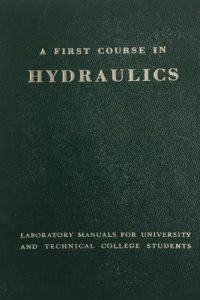اسم المؤلف
John D. Fenton
التاريخ
17 يونيو 2022
المشاهدات
121
التقييم(لا توجد تقييمات)Loading...

A First Course in Hydraulics
John D. Fenton
Institute of Hydraulic Engineering and Water Resources Management
Vienna University of Technology, Karlsplatz 13/222,
1040 Vienna, Austria
References . 4

1. The nature and properties of fluids, forces, and flows 5
1.1 Properties of fluids . 5
1.2 Forces acting on a fluid . 12
1.3 Units . 12
1.4 Turbulent flow and the nature of most flows in hydraulics . 12
2. Hydrostatics . 22
2.1 Fundamentals 22
2.2 Forces on submerged planar objects . 28
2.3 Forces on submerged boundaries of general shape . 31
2.4 The buoyancy and stability of submerged and floating bodies 36
3. Fluid kinematics and flux of quantities . 41
3.1 Kinematic definitions 41
3.2 Flux of volume, mass, momentum and energy across a surface . 42
3.3 Control volume, control surface . 44
4. Conservation of mass – the continuity equation 44
5. Conservation of momentum and forces on bodies . 45
1A First Course in Hydraulics John D. Fenton
6. Conservation of energy . 50
6.1 The energy equation in integral form . 50
6.2 Application to simple systems . 52
6.3 Bernoulli’s equation along a streamline 54
6.4 Crocco’s law – a generalisation of Bernoulli’s law . 56
6.5 Irrotational flow . 58
6.6 Summary of applications of the energy equation 59
7. Dimensional analysis and similarity . 59
7.1 Dimensional homogeneity 59
7.2 Buckingham Π theorem . 59
7.3 Useful points 60
7.4 Named dimensionless parameters 62
7.5 Physical scale modelling for solving flow problems . 63
8. Flow in pipes 64
8.1 The resistance to flow 64
8.2 Practical single pipeline design problems 68
8.3 Minor losses . 69
8.4 Pipeline systems . 71
9. Discharge measurement in pipes 86
9.1 Differential head meters – Venturi, nozzle and orifice meters 86
9.2 Acoustic flow meters 89
9.3 Magnetic Flowmeters 90
10. Pressure surges in pipes . 91
10.1 Introduction . 91
10.2 The phenomenon of water hammer 91
10.3 Sequence of events following sudden valve closure . 92
10.4 Derivation of fundamental relationship using steady momentum
theorem 93
10.5 Practical considerations . 94
11. Pumps and turbines 95
11.1 Introduction to pump types 95
11.2 Hydraulic ram pumps 96
11.3 Centrifugal Pumps . 97
11.4 Pump Performance Curves/Characteristic Curves . 98
11.5 Dimensional analysis 99
11.6 Similarity laws . 101
11.7 Series and parallel operation . 103
11.8 Euler equation for a rotor (impeller) 103
11.9 Water turbines .

كلمة سر فك الضغط : books-world.net